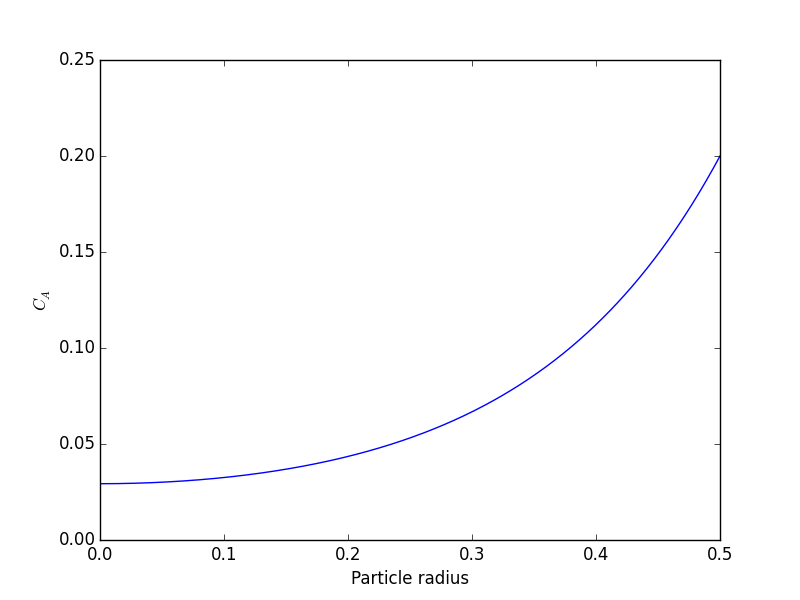## Numerically calculating an effectiveness factor for a porous catalyst bead

| categories: bvp | tags: reaction engineering

If reaction rates are fast compared to diffusion in a porous catalyst pellet, then the observed kinetics will appear to be slower than they really are because not all of the catalyst surface area will be effectively used. For example, the reactants may all be consumed in the near surface area of a catalyst bead, and the inside of the bead will be unutilized because no reactants can get in due to the high reaction rates.

References: Ch 12. Elements of Chemical Reaction Engineering, Fogler, 4th edition.

A mole balance on the particle volume in spherical coordinates with a first order reaction leads to:with boundary conditionsandat. We convert this equation to a system of first order ODEs by letting. Then, our two equations become:andWe have a condition of no flux () at r=0 and Ca(R) = CAs, which makes this a boundary value problem. We use the shooting method here, and guess what Ca(0) is and iterate the guess to get Ca(R) = CAs.

The value of the second differential equation at r=0 is tricky because at this place we have a 0/0 term. We use L'Hopital's rule to evaluate it. The derivative of the top isand the derivative of the bottom is 1. So, we haveorat.

Finally, we implement the equations in Python and solve.

import numpy as np
from scipy.integrate import odeint
import matplotlib.pyplot as plt

De = 0.1    # diffusivity cm^2/s
R = 0.5    # particle radius, cm
k = 6.4    # rate constant (1/s)
CAs = 0.2   # concentration of A at outer radius of particle (mol/L)

def ode(Y, r):
Wa = Y  # molar rate of delivery of A to surface of particle
Ca = Y  # concentration of A in the particle at r
# this solves the singularity at r = 0
if r == 0:
dWadr = k / 3.0 * De * Ca
else:
dWadr = -2 * Wa / r + k / De * Ca

# Initial conditions
Ca0 = 0.029315  # Ca(0) (mol/L) guessed to satisfy Ca(R) = CAs
Wa0 = 0         # no flux at r=0 (mol/m^2/s)

rspan = np.linspace(0, R, 500)

Y = odeint(ode, [Wa0, Ca0], rspan)

Ca = Y[:, 1]

# here we check that Ca(R) = Cas
print 'At r={0} Ca={1}'.format(rspan[-1], Ca[-1])

plt.plot(rspan, Ca)
plt.ylabel('$C_A$')
plt.savefig('images/effectiveness-factor.png')

r = rspan
eta_numerical = (np.trapz(k * Ca * 4 * np.pi * (r**2), r)
/ np.trapz(k * CAs * 4 * np.pi * (r**2), r))

print(eta_numerical)

phi = R * np.sqrt(k / De)
eta_analytical = (3 / phi**2) * (phi * (1.0 / np.tanh(phi)) - 1)
print(eta_analytical)

At r=0.5 Ca=0.200001488652
[<matplotlib.lines.Line2D object at 0x114275550>]
<matplotlib.text.Text object at 0x10d5fe890>
<matplotlib.text.Text object at 0x10d5ff890>
0.563011348314

0.563003362801You can see the concentration of A inside the particle is significantly lower than outside the particle. That is because it is reacting away faster than it can diffuse into the particle. Hence, the overall reaction rate in the particle is lower than it would be without the diffusion limit.

The effectiveness factor is the ratio of the actual reaction rate in the particle with diffusion limitation to the ideal rate in the particle if there was no concentration gradient:We will evaluate this numerically from our solution and compare it to the analytical solution. The results are in good agreement, and you can make the numerical estimate better by increasing the number of points in the solution so that the numerical integration is more accurate.

Why go through the numerical solution when an analytical solution exists? The analytical solution here is only good for 1st order kinetics in a sphere. What would you do for a complicated rate law? You might be able to find some limiting conditions where the analytical equation above is relevant, and if you are lucky, they are appropriate for your problem. If not, it is a good thing you can figure this out numerically!

Thanks to Radovan Omorjan for helping me figure out the ODE at r=0!